Home
Hostname: page-component-55597f9d44-l69ms Total loading time: 0.307 Render date: 2022-08-18T12:03:24.378Z Has data issue: true Feature Flags: { "shouldUseShareProductTool": true, "shouldUseHypothesis": true, "isUnsiloEnabled": true, "useRatesEcommerce": false, "useNewApi": true } hasContentIssue true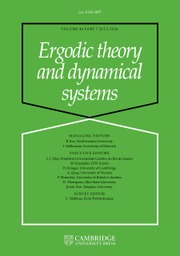Ergodic Theory and Dynamical Systems

# Oscillation and the mean ergodic theorem for uniformly convex Banach spaces

Published online by Cambridge University Press:  10 January 2014

## Abstract

Let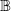$\mathbb{B}$ be a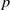$p$-uniformly convex Banach space, with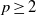$p\geq 2$. Let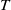$T$ be a linear operator on$\mathbb{B}$, and let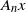${A}_{n} x$ denote the ergodic average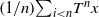$(1/ n){\mathop{\sum }\nolimits}_{i\lt n} {T}^{n} x$. We prove the following variational inequality in the case where$T$ is power bounded from above and below: for any increasing sequence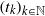$\mathop{({t}_{k} )}\nolimits_{k\in \mathbb{N} }$ of natural numbers we have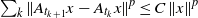${\mathop{\sum }\nolimits}_{k} \mathop{\Vert {A}_{{t}_{k+ 1} } x- {A}_{{t}_{k} } x\Vert }\nolimits ^{p} \leq C\mathop{\Vert x\Vert }\nolimits ^{p}$, where the constant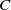$C$ depends only on$p$ and the modulus of uniform convexity. For$T$ a non-expansive operator, we obtain a weaker bound on the number of$\varepsilon$-fluctuations in the sequence. We clarify the relationship between bounds on the number of$\varepsilon$-fluctuations in a sequence and bounds on the rate of metastability, and provide lower bounds on the rate of metastability that show that our main result is sharp.

Type
Research Article
Information
Ergodic Theory and Dynamical Systems , June 2015 , pp. 1009 - 1027

## Access options

Get access to the full version of this content by using one of the access options below. (Log in options will check for institutional or personal access. Content may require purchase if you do not have access.)
5
Cited by

# Save article to Kindle

Note you can select to save to either the @free.kindle.com or @kindle.com variations. ‘@free.kindle.com’ emails are free but can only be saved to your device when it is connected to wi-fi. ‘@kindle.com’ emails can be delivered even when you are not connected to wi-fi, but note that service fees apply.

Find out more about the Kindle Personal Document Service.

Oscillation and the mean ergodic theorem for uniformly convex Banach spaces
Available formats
×

# Save article to Dropbox

To save this article to your Dropbox account, please select one or more formats and confirm that you agree to abide by our usage policies. If this is the first time you used this feature, you will be asked to authorise Cambridge Core to connect with your Dropbox account. Find out more about saving content to Dropbox.

Oscillation and the mean ergodic theorem for uniformly convex Banach spaces
Available formats
×

# Save article to Google Drive

To save this article to your Google Drive account, please select one or more formats and confirm that you agree to abide by our usage policies. If this is the first time you used this feature, you will be asked to authorise Cambridge Core to connect with your Google Drive account. Find out more about saving content to Google Drive.

Oscillation and the mean ergodic theorem for uniformly convex Banach spaces
Available formats
×
×